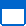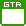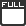#### Mastering DAX

Length: 3 Day(s)     Cost:\$2100 + GST= Scheduled class= Guaranteed to run= Fully booked

Click on the date to book online
LOCATION June July August September
Auckland
Hamilton
Christchurch
Wellington
Virtual Class

The goal of the course is to demonstrate all the features of DAX, providing the knowledge to write formulas for common and advanced business scenarios. Students will use DAX to solve real world scenarios that enhance reporting capabilities.

While DAX can be used across a variety of applications, this course will be taught using Power BI.

Recently extended to a 3 day course to include columns and measures, context transition, variables and optimization.

Have you been using DAX for a while? Are you drowning in Youtube videos? Tired of copying DAX expressions that you don’t understand? This training is aimed at Power BI, Power Pivot for Excel and Analysis Services developers who wish to gain a better understanding of DAX and the context behind it. Discover some of the lesser-known DAX functions, explore the different behaviours of similar functions (and discuss when to use each) and master your understanding of CALCULATE.

Before attending this course you must:

• have experience with Power BI equivalent to that covered in Power BI Essential Skills
• understand reporting data models in Power BI, Excel PowerPivot or SSASS Tabular Model
• have a basic understanding of DAX functions and syntax (ie able to use SUM, CALCULATE, or IF functions to create calculated columns and measures)

Upon completion of this course, students will:

• Create complex calculated measures to further extend the reporting capabilities of the Power BI data model
• Understand the context of the DAX language and how it works within the report’s data model
• Demonstrate proper use of the CALCULATE function and how it behaves
• Use table functions to dynamically filter measures
• Understand the properties of a Date table and use it to create time intelligence measures
• Know what to look out for when using DAX in reporting

Module 1: Introduction to DAX

A review of DAX and its most basic functions; SUM, MIN, MAX. In this module, you will get familiar with the dataset that we will be using throughout the course and learn a few tricks for keeping your DAX code easy to read.

Topics:

What is DAX?

DAX Syntax

DAX Functions

DAX Operators

Data Types

Common DAX Functions

Labs:

Unit Price Analysis

Reporting Sales

Module 2: Columns vs Measures

Learn the differences between calculated columns and measures, where to use each, when they are calculated, and how they are impacted by slicers and filters in your report. Learn how to leverage relationships in your data model to create calculated columns using data from multiple tables.

Calculated Columns

Implicit vs Explicit Measures

Naming Convention

Using measures and columns

Labs:

Sale Type by City

Discounts

Module 3: Introduction to Evaluation Contexts

Now that you understand columns vs measures, start to debunk the concept of Evaluation Contexts in DAX and understand why measures behave the way they do.

Introduction to evaluation contexts

Filter Context

Row Context

Labs:

Average Sales

Module 4: Introduction to CALCULATE

CALCULATE is a powerful DAX function that is often misunderstood. Learn the basic syntax of CALCULATE and use it to calculate ratios.

Topics:

Introduction to CALCULATE

CALCULATE modifiers

Labs:

Ratios and Percentages

Module 5: Context Transition

In this module, we will continue working with the CALCULATE function and explore its power of context transition. Learn what this means for your calculated columns.

Topics:

Introduction to Context transition

Automatic CALCULATE in measures

Equivalent Filter context

Labs:

Manipulating Evaluations Contexts

Module 6: Working with Iterators

Iterators create a virtual calculated column. This can help reduce the amount of DAX you need to write to get your desired result. In this module, we’ll explore common iterators and see the importance of understanding that evaluation context when working with these virtual calculated columns.

Topics:

Introduction to Iterators

Useful iterators

Aggregating Expressions

Labs:

Sales per Working Day

Module 7: Table Filter Functions

Now that you’re familiar with Iterators, we can leverage the power of table filter functions to narrow in on exactly the information we need.

Topics:

Introduction to Table Functions

FILTER Function

VALUES, DISTINCT Functions

Labs:

Ranking Stores by Profit

Finding above average Customers

Module 8: Evaluation contexts and Relationships

Building the right data model is crucial to the success of any report. Learn how DAX uses the relationships in your data model to calculate the results.

Topics:

Row context and relationships

Filter context and relationships

Filtering many columns

Cross filtering

Expanded tables

USERELATIONSHIP

Labs:

Total Shipments vs Total Orders

Module 9: Variables

Variables can help keep your DAX code tidy and optimized, but more importantly they can provide context transition within measures. In this module, we will learn how variables are calculated and when to use them.

Topics:

Variables syntax

Calculation of variables

Variables for optimization

Variables for context transition

EARLIER function vs variables

Labs:

First purchase amount

Module 10: Date Table

Having a robust date table is key to any time intelligence calculations. Learn the few simple steps you need to take to ensure your time intelligence functions calculate properly.

Topics:

Date table properties

CALENDAR vs CALENDARAUTO vs import

Multiple dates

Labs:

Mark as Date Table

Set sorting options

Module 11: Time Intelligence

Don’t break out the calculator just yet- DAX time intelligence functions enable you to easily make comparisons for Year on Year, Month on Month, Year to Date, 12 Month Rolling average and more. In this module, we will learn the formula for success with DAX time intelligence.

Topics:

Introduction to Time Intelligence

Common Time Intelligence Functions

Time Intelligence DAX formula for success

Labs:

Year to Date Sales Percent Change (from previous year)

Rolling 12 Month Average

Module 12: Optimization

Now that know all the common DAX functions and their evaluation contexts, how do you optimize your code? In this module, we will look at some tools for determining the performance of your DAX as well as some tips to help improve that performance.

Topics:

Performance analyzer

Variables

Naming conventions

Labs:

Percent growth calculated two ways